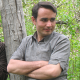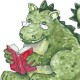# Math is not Science!Occupy Math is a member of the College of Physical and Engineering Sciences at the University of Guelph. The fact that the Department of Mathematics and Statistics is in this college makes it seem as if mathematics is one of the sciences — but it is not. Math is often considered to be part of the natural sciences, and it is central to and remarkably useful to the natural sciences, but the techniques, methods, and philosophy of math are different from those of natural science. Technically, based on its techniques, mathematics is the most extreme of the humanities.

The center of the natural sciences is hypothesis-driven research and experimentation. Experiments yield evidence that supports or fails to support a hypothesis. When new information arrives from experiments, a hypothesis may be revised. Math does exactly none of this. In math, a statement is shown to be true or false by logical argumentation. This means that there is no doubt once a proof of the truth or falsity of a mathematical statement is finished. The methods of mathematics are so different from those of the natural sciences that it is clearly a different sort of animal.

Why do people think math is a science?

The short version of the answer is because (i) many scientists use a lot of math and (ii) a whole lot of math was discovered in order to answer a scientific question. Someone once asked Sir Isaac Newton if the mass of a planet could be considered to be concentrated in the center of mass. He answered in the affirmative — and in order to do so, he invented at least part of integral calculus.

Both scientists and mathematicians perform extensive calculations. This makes them seem similar to people. One of the most mathematical fields, however, is economics — one of the humanities. One of the primary tools that mathematicians use in proving things is formal logic. Formal logic is one of the fields within philosophy — another humanity. Alfred North Whitehead and Bertrand Russell tried to prove that all of math could be deduced by first order logic. Gödel’s incompleteness theorem showed this was not possible — but the proof Gödel constructed illustrates that mathematics is more similar to formal linguistics than any of the natural sciences.

The contrast between math and science.

A new piece of mathematics starts with a guess at something that might be true, called a conjecture. Some conjectures are resolved quickly by the person that proposed them, others last for centuries. Fermat’s last theorem, for example, was actually a conjecture for over 350 years, until it was finally proved in 1995. Contrast this with the Law of Universal Gravitation. This law was never proved logically — rather, it agrees very well with numerous observations. It’s also slightly wrong in some odd circumstances.

Proven mathematical theorems are beyond question. The laws discovered by science are really good approximations that are often false in some circumstances or in small ways. The certainty of math arises from its completely abstract nature. A mathematical theorem never makes a statement about reality. A theorem connects a statement about what the situation is to a result of that situation. The fundamental theorem of arithmetic says every whole number factors into prime numbers in exactly one way, as long as we ignore the order of those factors. Whole numbers are abstractions. We use them to count things, but we use our own human notion of “thing” to do it.

Except…

…there is such a thing as experimental mathematics. The methods of science are powerful and can reach farther, in some directions, that the methods of mathematics. The first step in making new math is a conjecture and experiments are a good way to find conjectures that are likely to be true. Occupy Math’s own doctoral thesis used a series of experiments — none of which appeared in the final document. The experiments suggested how to build a whole series of secret codes and also what their structure was. This suggestion led to a conjecture — and (nine months later) to a proof about the structure of the codes — that showed all of them were easy to crack. At the time Occupy Math was in graduate school, reporting the experiments would have been socially unacceptable.

Most of the things that science works on are much too hard to figure out using only the techniques of mathematics. Many of the things that mathematics figures out are unrelated to physical reality. The relationship between math and science is symbiotic. Science views math as an important source of tools, math views science as a wonderful source of examples. Beyond this, the needs of science often spark the discovery of new mathematics and, occasionally, math worked out in a completely abstract setting turns out to be useful for science. Some of the topology that describes string theory, for example, is older than the idea of string theory.

Occupy Math has read, listened to, and participated in many discussions about the relationship between math and science. His view, that they are symbiotic but different, is the result of long cogitation on the issue. If you have your own thoughts on this, please comment!

I hope to see you here again,
Daniel Ashlock,
University of Guelph,
Department of Mathematics and Statistics

## 4 thoughts on “Math is not Science!”

1.goldenoj says:

Every time I teach our capstone, Nature of Mathematics, which I do with a history focus, the learners seem to gravitate towards a big question. Invented or discovered? Is math a language? Or, this one, which I love, is math a science? (OK, I love all of them.) This question really gets them focused on what mathematicians do. It seems to come down to yes vs math is a tool scientists use.

For me, I think science admits a variety of methodologies and purposes, so I like to think of math as a science… maybe even the Queen of the Sciences. (Half of the Gauss quotation.) To some extent, I think it maybe inspired some of the other sciences by having things that were always true, and reasoning that invites causality.

But I’ll probably comment on the post asking whether math is an art with a yes to that, too.

Like

2.Samuel says:

I really enjoyed your article. you have properly answered to one of the really old questions around math. I have so many times experienced the act of explaining that math is more independent than be a result of engineering or natural sciences. I sometimes think it’s better to accept math a language! not a science. as a novelist create a story by using some language, a mathematician also create a story: both describe human’s life very good but they are far way to be only a translation of reality.
any way thank you so much
best regards
Samuel ABRAHAMIAN

Liked by 1 person

3.sue clancy says:

I’ve often thought that math and fine art had a lot of thinking/methodologies in common …. hummm… 🙂

Like